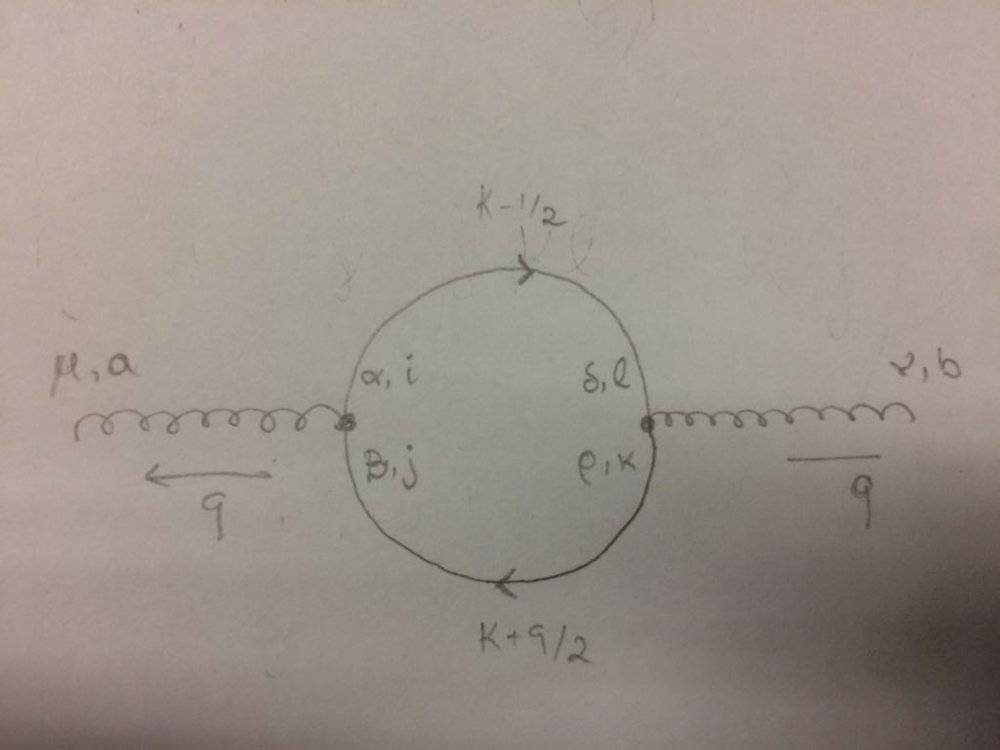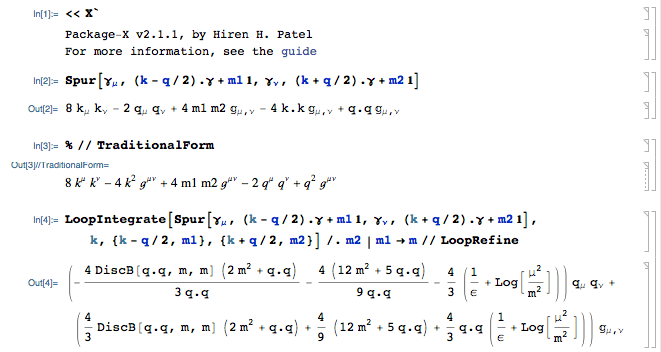# Trace of a product of Dirac Matrices in a Fermion loop

• A
I'm working out the quark loop diagram and I've drawn it as follows:where the greek letters are the Lorentz and Dirac indices for the gluon and quark respectively and the other letters are color indices.
For this diagram I've written:

$$i\Pi^{\mu\nu}=\int_{}^{}\frac{d^4k}{(2\pi)^4}.[ig(t_a)_{ij}\gamma^\mu].[\frac{i[(\not\! k-\frac{\not{q}}{2})+m_1]}{(k-\frac{q}{2})^2-m_1+i\epsilon}\delta_{il}].[ig(t_b)_{kl}\gamma^\nu].[\frac{i[(\not\! k+\frac{\not{q}}{2})+m_2]}{(k+\frac{q}{2})^2-m_2+i\epsilon}\delta_{kj}]$$

In Peskin & Schroeder's Introduction to QFT it is said: "a closed fermion loop always gives a factor of -1 and the trace of a product of Dirac matrices". In short, I can write the above expression as:

$$i\Pi^{\mu\nu}=g^2T_F\delta{ab}\int_{}^{}\frac{d^4k}{(2\pi)^4}\frac{tr[\gamma^\mu.((\not\! k-\frac{\not{q}}{2})+m_1).\gamma^\nu.((\not\! k+\frac{\not{q}}{2})+m_2)]}{((k-\frac{q}{2})^2-m_1+i\epsilon).((k+\frac{q}{2})^2-m_2+i\epsilon)}$$

where ##T_F## is the Dynkin index in the fundamental representation I get after contracting the generators and deltas.
What I'm having a hard time with is on how to explicitly write the Dirac indices in each gamma matrix and slashed momenta in order to get something of the sort, e.g, ##(matrix)_{ii}=tr(matrix)##.

Orodruin
Staff Emeritus
Homework Helper
Gold Member
What is tr(AB) in terms of the components of A and B?

Also, I am unsure why you would want to write this out explicitly. It is far (far!) easier to use gamma matrix and trace relations to compute the trace.

vanhees71
Gold Member
There are some useful formulae for such traces in the appendix of Peskin&Schroeder. You can derive them by using the anticommutation relations of the Dirac matrices.

What is tr(AB) in terms of the components of A and B?

Also, I am unsure why you would want to write this out explicitly. It is far (far!) easier to use gamma matrix and trace relations to compute the trace.
I might have not been clear, I'm sorry. I do want to use the trace identities in order to do the calculations. I just wanted to write out the indices explicitly so I show clearly that the numerator is indeed a trace.

vanhees71
Gold Member
First the matrix product is (Einstein summation convention used)
$$(AB)_{ij}=A_{ik} B_{kj}$$
Then the trace is the sum of the diagonal elements. In the Ricci calculus you just have to set ##i=j## in the above formula (implying summation over ##i## then of course):
$$\mathrm{Tr}(AB)=A_{ik} B_{ki}.$$

I have written a Mathematica program called Package-X that can help you with your Dirac traces and loop calculation. I suggest after completing the calculation by hand, you check it with the output of Package-X.

Here is what the calculation might look like in your Mathematica notebook.Copy the following into a blank Mathematica notebook for editable code:
Trace-PackageX.nb:
Notebook[{

Cell[CellGroupData[{
Cell[BoxData[
RowBox[{"<<", "X"}]], "Input",
CellLabel->"In:=",ExpressionUUID->"d68acd69-9e6f-4b16-be40-73037f22b7d6"],

Cell[BoxData["\<\"\\!\$$\\*TemplateBox[List[\\\"\\\\\\\"Package-X v2.1.1, by \ Hiren H. Patel\\\\\\\\nFor more information, see the \\\\\\\"\\\", \ TemplateBox[List[\\\"\\\\\\\"guide\\\\\\\"\\\", \\\"paclet:X/guide/PackageX\\\ \"], \\\"HyperlinkPaclet\\\"]], \\\"RowDefault\\\"]\$$\"\>"], "Print",
CellLabel->
"During evaluation of \
In:=",ExpressionUUID->"befefef7-2330-4488-a63e-82744427c722"]
}, Open  ]],

Cell[CellGroupData[{

Cell[BoxData[
RowBox[{"Spur", "[",
RowBox[{
SubscriptBox["\[Gamma]", "\[Mu]"], ",",
RowBox[{
RowBox[{
RowBox[{"(",
RowBox[{"k", "-",
RowBox[{"q", "/", "2"}]}], ")"}], ".", "\[Gamma]"}], "+",
RowBox[{"m1", " ", "\[DoubleStruckOne]"}]}], ",",
SubscriptBox["\[Gamma]", "\[Nu]"], ",",
RowBox[{
RowBox[{
RowBox[{"(",
RowBox[{"k", "+",
RowBox[{"q", "/", "2"}]}], ")"}], ".", "\[Gamma]"}], "+",
RowBox[{"m2", " ", "\[DoubleStruckOne]"}]}]}], "]"}]], "Input",
CellLabel->"In:=",ExpressionUUID->"c1fd717f-9d65-4d47-8be3-7152b8684d0b"],

Cell[BoxData[
RowBox[{
RowBox[{"8", " ",
SubscriptBox["k", "\[Mu]"], " ",
SubscriptBox["k", "\[Nu]"]}], "-",
RowBox[{"2", " ",
SubscriptBox["q", "\[Mu]"], " ",
SubscriptBox["q", "\[Nu]"]}], "+",
RowBox[{"4", " ", "m1", " ", "m2", " ",
SubscriptBox["\[DoubleStruckG]",
RowBox[{"\[Mu]", ",", "\[Nu]"}]]}], "-",
RowBox[{"4", " ",
RowBox[{"k", ".", "k"}], " ",
SubscriptBox["\[DoubleStruckG]",
RowBox[{"\[Mu]", ",", "\[Nu]"}]]}], "+",
RowBox[{
RowBox[{"q", ".", "q"}], " ",
SubscriptBox["\[DoubleStruckG]",
RowBox[{"\[Mu]", ",", "\[Nu]"}]]}]}]], "Output",
CellLabel->"Out=",ExpressionUUID->"c7080a80-df67-433a-9f81-e826e245e4c9"]
}, Open  ]],

Cell[CellGroupData[{

Cell[BoxData[
CellLabel->"In:=",ExpressionUUID->"a01137ae-5895-4b17-bb2d-77e1b5d0a83c"],

Cell[BoxData[
FormBox[
RowBox[{
RowBox[{"8", " ",
SuperscriptBox["k", "\[Mu]"], " ",
SuperscriptBox["k", "\[Nu]"]}], "-",
RowBox[{"4", " ",
SuperscriptBox["k", "2"], " ",
SuperscriptBox["\[ScriptG]",
RowBox[{"\[Mu]", "\[InvisibleComma]", "\[Nu]"}]]}], "+",
RowBox[{"4", " ", "m1", " ", "m2", " ",
SuperscriptBox["\[ScriptG]",
RowBox[{"\[Mu]", "\[InvisibleComma]", "\[Nu]"}]]}], "-",
RowBox[{"2", " ",
SuperscriptBox["q", "\[Mu]"], " ",
SuperscriptBox["q", "\[Nu]"]}], "+",
RowBox[{
SuperscriptBox["q", "2"], " ",
SuperscriptBox["\[ScriptG]",
RowBox[{"\[Mu]", "\[InvisibleComma]", "\[Nu]"}]]}]}],
CellLabel->
3f50f76001f2"]
}, Open  ]],

Cell[CellGroupData[{

Cell[BoxData[
RowBox[{
RowBox[{
RowBox[{"LoopIntegrate", "[",
RowBox[{
RowBox[{"Spur", "[",
RowBox[{
SubscriptBox["\[Gamma]", "\[Mu]"], ",",
RowBox[{
RowBox[{
RowBox[{"(",
RowBox[{"k", "-",
RowBox[{"q", "/", "2"}]}], ")"}], ".", "\[Gamma]"}], "+",
RowBox[{"m1", " ", "\[DoubleStruckOne]"}]}], ",",
SubscriptBox["\[Gamma]", "\[Nu]"], ",",
RowBox[{
RowBox[{
RowBox[{"(",
RowBox[{"k", "+",
RowBox[{"q", "/", "2"}]}], ")"}], ".", "\[Gamma]"}], "+",
RowBox[{"m2", " ", "\[DoubleStruckOne]"}]}]}], "]"}], ",", "k", ",",
RowBox[{"{",
RowBox[{
RowBox[{"k", "-",
RowBox[{"q", "/", "2"}]}], ",", "m1"}], "}"}], ",",
RowBox[{"{",
RowBox[{
RowBox[{"k", "+",
RowBox[{"q", "/", "2"}]}], ",", "m2"}], "}"}]}], "]"}], "/.",
RowBox[{
RowBox[{"m2", "|", "m1"}], "\[Rule]", "m"}]}], "//",
"LoopRefine"}]], "Input",
CellLabel->"In:=",ExpressionUUID->"26c2c3b2-c443-45d6-985b-d7d5004495c0"],

Cell[BoxData[
RowBox[{
RowBox[{
RowBox[{"(",
RowBox[{
RowBox[{"-",
FractionBox[
RowBox[{"4", " ",
RowBox[{"DiscB", "[",
RowBox[{
RowBox[{"q", ".", "q"}], ",", "m", ",", "m"}], "]"}], " ",
RowBox[{"(",
RowBox[{
RowBox[{"2", " ",
SuperscriptBox["m", "2"]}], "+",
RowBox[{"q", ".", "q"}]}], ")"}]}],
RowBox[{"3", " ",
RowBox[{"q", ".", "q"}]}]]}], "-",
FractionBox[
RowBox[{"4", " ",
RowBox[{"(",
RowBox[{
RowBox[{"12", " ",
SuperscriptBox["m", "2"]}], "+",
RowBox[{"5", " ",
RowBox[{"q", ".", "q"}]}]}], ")"}]}],
RowBox[{"9", " ",
RowBox[{"q", ".", "q"}]}]], "-",
RowBox[{
FractionBox["4", "3"], " ",
RowBox[{"(",
RowBox[{
FractionBox["1", "\[Epsilon]"], "+",
RowBox[{"Log", "[",
FractionBox[
SuperscriptBox["\[Micro]", "2"],
SuperscriptBox["m", "2"]], "]"}]}], ")"}]}]}], ")"}], " ",
SubscriptBox["q", "\[Mu]"], " ",
SubscriptBox["q", "\[Nu]"]}], "+",
RowBox[{
RowBox[{"(",
RowBox[{
RowBox[{
FractionBox["4", "3"], " ",
RowBox[{"DiscB", "[",
RowBox[{
RowBox[{"q", ".", "q"}], ",", "m", ",", "m"}], "]"}], " ",
RowBox[{"(",
RowBox[{
RowBox[{"2", " ",
SuperscriptBox["m", "2"]}], "+",
RowBox[{"q", ".", "q"}]}], ")"}]}], "+",
RowBox[{
FractionBox["4", "9"], " ",
RowBox[{"(",
RowBox[{
RowBox[{"12", " ",
SuperscriptBox["m", "2"]}], "+",
RowBox[{"5", " ",
RowBox[{"q", ".", "q"}]}]}], ")"}]}], "+",
RowBox[{
FractionBox["4", "3"], " ",
RowBox[{"q", ".", "q"}], " ",
RowBox[{"(",
RowBox[{
FractionBox["1", "\[Epsilon]"], "+",
RowBox[{"Log", "[",
FractionBox[
SuperscriptBox["\[Micro]", "2"],
SuperscriptBox["m", "2"]], "]"}]}], ")"}]}]}], ")"}], " ",
SubscriptBox["\[DoubleStruckG]",
RowBox[{"\[Mu]", ",", "\[Nu]"}]]}]}]], "Output",
CellLabel->"Out=",ExpressionUUID->"b84b5d35-33bc-4be1-97fe-7f6dcc187173"]
}, Open  ]]
},
WindowSize->{676, 876},
WindowMargins->{{Automatic, 626}, {Automatic, 156}},
FrontEndVersion->"12.0 for Mac OS X x86 (64-bit) (April 8, 2019)",
StyleDefinitions->"Default.nb"
]`